# Methods and formulas for fits and residuals in Partial Least Squares Regression

Select the method or formula of your choice.

## Fitted values

The predicted Y or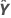is the mean response value for the given predictor values using the estimated regression equation.

## Cross-validated fitted values

Cross-validated fitted values indicate how well your model predicts data. These values are similar to ordinary fitted values, which indicate how well your model fits the data. To obtain cross-validated fitted value for an observation, it must be removed from the data used to calculate the model and then the fit is calculated with the coefficient vector that is independent from the observation. The formula for the cross-validated fitted values is as follows:

### Notation

TermDescription
\iIndicates that i observation was left out of the model calculation
b0\ithe intercept for the model that does not include observation i
Xthe predictor values
B(\i)(j, k) the coefficients for the model that does not include observation i

## Residuals

The residual is the difference between an observed value and the corresponding fitted value. This part of the observation is not explained by the model. The residual of an observation is:

### Notation

TermDescription
yiith observed response valueith fitted value for the response

## Cross-validated residuals

Cross-validated residuals measure the model's predictive ability and are used to calculate the PRESS statistic. Cross-validated residuals in PLS and least squares regression are conceptually similar, but their calculations differ.

### Formula

In PLS, the cross-validated residuals are the differences between the actual responses and the cross-validated fitted values.

The cross-validated residual value varies based on how many observations are omitted each time the model is recalculated during cross-validation.

In least squares regression, the cross-validated residuals are calculated directly from the ordinary residuals.

### Notation

TermDescription
(i) observation omitted from the model calculation
yi response value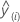cross-validated fitted value

## Standardized residual (Std Resid)

Standardized residuals are also called "internally Studentized residuals."

### Notation

TermDescription
ei i th residual
hi i th diagonal element of X(X'X)–1X'
s2 mean square error
Xdesign matrix
X'transpose of the design matrix

## Standard error of fitted value (SE Fit)

The standard error of the fitted value in a regression model with one predictor is:

The standard error of the fitted value in a regression model with more than one predictor is:

### Notation

TermDescription
s2mean square error
nnumber of observations
x0new value of the predictor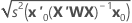mean of the predictor
xiith predictor value
x0 vector of values that produce the fitted values, one for each column in the design matrix, beginning with a 1 for the constant term
x'0transpose of the new vector of predictor values
Xdesign matrix

## Confidence interval

The confidence interval is the range in which the estimated mean response for a given set of predictor values is expected to fall. The interval is defined by lower and upper limits, which Minitab calculates from the confidence level and the standard error of the fits.

### Notation

TermDescription
α alpha value
n number of observations
p number of predictors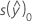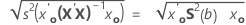s 2 mean square error
S 2(b)variance-covariance matrix of the coefficients

## Prediction interval

The prediction interval is the range in which the fitted response for a new observation is expected to fall.

### Notation

TermDescription
s(Pred)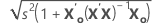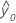fitted response value for a given set of predictor values
α level of significance
n number of observations
p number of model parameters
s 2 mean square error
X predictor matrix
X0 matrix of given predictor values
X'0transpose of the new vector of predictor values
By using this site you agree to the use of cookies for analytics and personalized content.  Read our policy